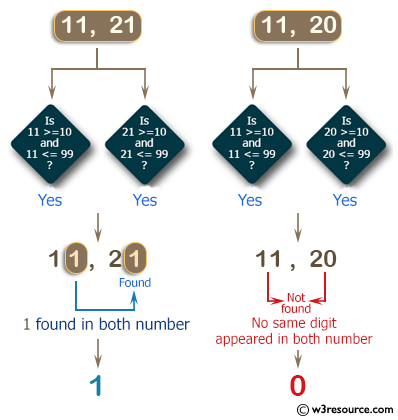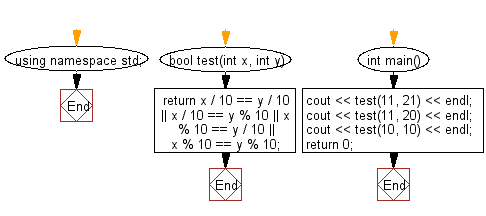﻿ C++ : Check two given integers, each in the range 10..99# C++ Exercises: Check two given integers, each in the range 10..99

## C++ Basic Algorithm: Exercise-50 with Solution

Write a C++ program to check two given integers, each in the range 10..99. Return true if a digit appears in both numbers, such as the 3 in 13 and 33.

Sample Solution:

C++ Code :

``````#include <iostream>
using namespace std;

bool test(int x, int y)
{
return x / 10 == y / 10 || x / 10 == y % 10 || x % 10 == y / 10 || x % 10 == y % 10;
}

int main()
{
cout << test(11, 21) << endl;
cout << test(11, 20) << endl;
cout << test(10, 10) << endl;
return 0;
}
``````

Sample Output:

```1
0
1
```

Pictorial Presentation:Flowchart:C++ Code Editor: